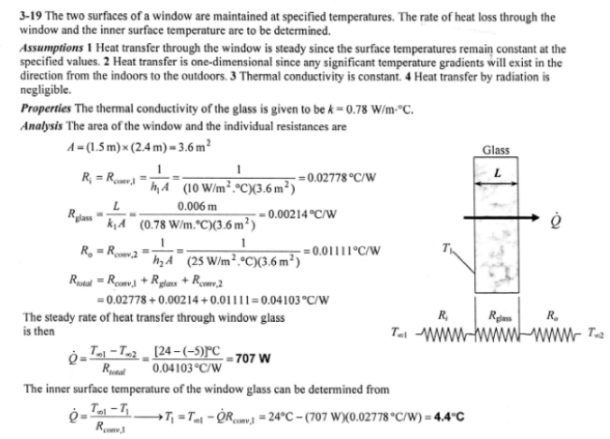heat and mass transfer problems engineering equations heat and mass transfer school homework engineering heat and mass transfer formulas heat and mass transfer solutions to heat and mass transfer problems full solution engineering problem solution heat and mass transfer math problems engineering equations heat and mass transfer school homework engineering solutions to heat and mass transfer formulas heat problems mass problem solutions to transfer problems full solution heat and mass transfer
heat and mass transfer problems engineering equations heat and mass transfer school homework engineering heat and mass transfer formulas heat and mass transfer solutions to heat and mass transfer problems full solution engineering problem solution heat and mass transfer math problems engineering equations heat and mass transfer school homework engineering solutions to heat and mass transfer formulas heat problems mass problem solutions to transfer problems full solution heat and mass transfer
Highalphabet Home Page heat and mass transfer problem solutions Heat and Mass Transfer Page
Consider a 1.5-m-high and 2.4-m-wide glass window whose thickness is 6 mm and thermal conductivity is k=0.78 W/mK. Determine the steady rate of heat transfer through this glass window and the temperature of its inner surface for a day during which the room is maintained at 24 C while the temperature of the outdoors is -5 C. Take the convection heat transfer coefficients on the inner and outer surfaces of the window to be 10 W/m^2K and 25 W/m^2K, and disregard any heat transfer by radiation.Consider a 1.5-m-high and 2.4-m-wide glass window whose thickness is 6 mm and thermal conductivity is k=0.78 W/mK. Determine the steady rate of heat transfer through this glass window and the temperature of its inner surface for a day during which the room is maintained at 24 C while the temperature of the outdoors is -5 C. Take the convection heat transfer coefficients on the inner and outer surfaces of the window to be 10 W/m^2K and 25 W/m^2K, and disregard any heat transfer by radiation.